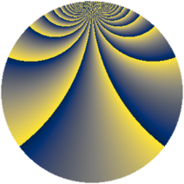# Properties

 Label 1148.2.yLevel $1148$ Weight $2$ Character orbit 1148.y Rep. character $\chi_{1148}(407,\cdot)$ Character field $\Q(\zeta_{8})$ Dimension $504$ Sturm bound $336$

# Learn more about

## Defining parameters

 Level: $$N$$ $$=$$ $$1148 = 2^{2} \cdot 7 \cdot 41$$ Weight: $$k$$ $$=$$ $$2$$ Character orbit: $$[\chi]$$ $$=$$ 1148.y (of order $$8$$ and degree $$4$$) Character conductor: $$\operatorname{cond}(\chi)$$ $$=$$ $$164$$ Character field: $$\Q(\zeta_{8})$$ Sturm bound: $$336$$

## Dimensions

The following table gives the dimensions of various subspaces of $$M_{2}(1148, [\chi])$$.

Total New Old
Modular forms 688 504 184
Cusp forms 656 504 152
Eisenstein series 32 0 32

## Trace form

 $$504q - 24q^{6} - 16q^{9} + O(q^{10})$$ $$504q - 24q^{6} - 16q^{9} - 8q^{12} + 56q^{17} + 8q^{29} - 32q^{30} + 40q^{32} - 80q^{33} - 40q^{34} + 24q^{36} + 40q^{41} - 80q^{42} + 40q^{52} - 8q^{53} + 72q^{54} + 40q^{60} - 40q^{61} - 72q^{62} - 152q^{74} - 104q^{78} - 80q^{80} + 32q^{85} + 8q^{88} - 16q^{89} - 152q^{92} - 200q^{94} - 128q^{96} - 40q^{97} + O(q^{100})$$

## Decomposition of $$S_{2}^{\mathrm{new}}(1148, [\chi])$$ into newform subspaces

The newforms in this space have not yet been added to the LMFDB.

## Decomposition of $$S_{2}^{\mathrm{old}}(1148, [\chi])$$ into lower level spaces

$$S_{2}^{\mathrm{old}}(1148, [\chi]) \cong$$ $$S_{2}^{\mathrm{new}}(164, [\chi])$$$$^{\oplus 2}$$